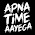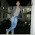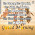## Tuesday, 29 November 2016

Consider Volume (Wet) = 1 m3
Grade of Concrete = M15 (Cement - 1 : sand - 2 : Aggregate - 4)
Sum Of Ratio = 1+2+4 = 7

Dry Volume = Wet Volume + 54% of Wet Volume = 1 + 0.54 = 1.54 m3

CEMENT
1. CUM : (1.54 x 1)/7 = 0.22 CUM
2. Kg : (1.54 x 1 x 1440)/7 = 316.8 Kg
3. Bags : We know 1 bag of cement weight = 50 Kg
Then, 316.8 Kg contains, 316.8/50 = 6.336 bags
4. CFT : If we multiply No. of bags by 1.226 then we get the value in CFT.
i.e, 6.336 x 1.226 = 7.77 CFT

SAND
1. CUM : (1.54 x 2)/7 = 0.44 CUM
2. Kg : (1.54 x 2 x 1450)/7 = 638 Kg [Density Varies in between 1450-1500 Kg/m3]
3. CFT : (1.54 x 2 x 35.3147)/7 = 15.54 CFT
Alternative : No. of Cement Bags x Value of 1 bag of cement in CFT x ratio of sand
= 6.336 x 1.226 x 2 = 15.54 CFT

AGGREGATE
1. CUM : (1.54 x 4)/7 = 0.88 CUM
2. Kg : (1.54 x 4 x 1500)/7 = 1320 Kg [Density Varies in between 1450-1550 Kg/m3]
3. CFT : (1.54 x 4 x 35.3147)/7 = 31.07 CFT
Alternative : No. of Cement Bags x Value of 1 bag of cement in CFT x ratio of sand
= 6.336 x 1.226 x 4 = 31.07 CFT

SHARE THIS POST, IF YOU LIKE IT !!
Padhega India Tab Hi Badhega India | पढ़ेगा इंडिया तब ही बढ़ेगा इंडिया

1.how much Quantity of water ?

1.2.w/c=.40
then 50*.40=20 ltr per bag of cement

2.3.4.Thanks.....

5.thank you , u r doing really good job sir

6.This comment has been removed by the author.

7.Thank you soo much sir....

8.Thank you soo much sir....

9.Mujhe pta nhi hai

10.Mujhe pta nhi hai

11.can ratio of dry water ratio change in other concret ratio means M25 etc

12.good work , keep going on it's very helpfull.

13.sir job ki requirement hai

14.15.sir girder ka bbs bataye please..

16.sir;M35 ya kisi v design mix k nikalna ho to kse nikalnge

17.This comment has been removed by the author.

18.sir, quantity of water?Widget